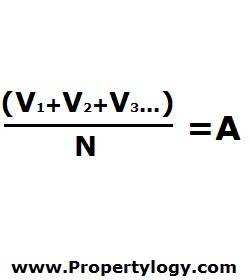Formula - Weighted Moving Average | Propertylogy
Don't Miss

# Formula – Weighted Moving Average

By on June 13, 2018

When the moving average is needed for investment analysis and the most recent data carries a lot more weight than the earlier ones, the weighted moving average would be a more appropriate formula to use.

There are various methods used to calculate moving average. But 2 of which are the most commonly used by business analysts.

1) Double the latest value and adding 1 to total values

If we take for example, the value is month end and the period is a year, there would be a total of 12 month end values to work with.(V+Vr)/(N+1) = A

V – Sum of values

Vr – Most recent value

N – Number of values

A – Weighted moving average

2) Use a reducing factor starting from the latest value

Using the previous scenario as example, the most recent value will multiply by 12, the second most recent multiply by 11, and so on until the last value.

Then divide the total sum of value by the number of value which is equivalent to 78. (12+11+10…)(V1+V2+V3…)/N = A

V1 – Most recent value, V2 second most recent value, and so on

N – Number of values

A – Weighted moving average

If you have your own variables that carry a greater weight, use your own methods to give more weight to more important values.

You May Also Like...##### How to burn more calories in the office

Send this to a friend